IntroductionIntroduction# Surface Area of Cubes and Rectangular Prisms

INTRO
The surface area of a 3D shape is equal to the sum of the areas of all the faces (sides) of that shape. A helpful way to think about it is:
surface area = sum of face areas
We can also think about surface area in terms of gifts! The surface area is like the minimum amount of wrapping paper we need to prep a gift for its surprise reveal. 🤩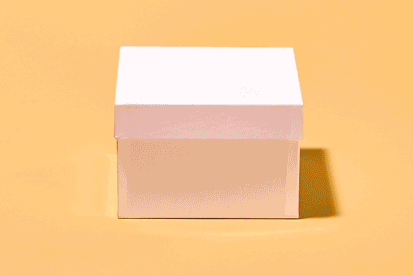If we were to unwrap a gift, the amount of wrapping would be equal to the surface area of the object it was covering.

Pick a gift shape to unwrap: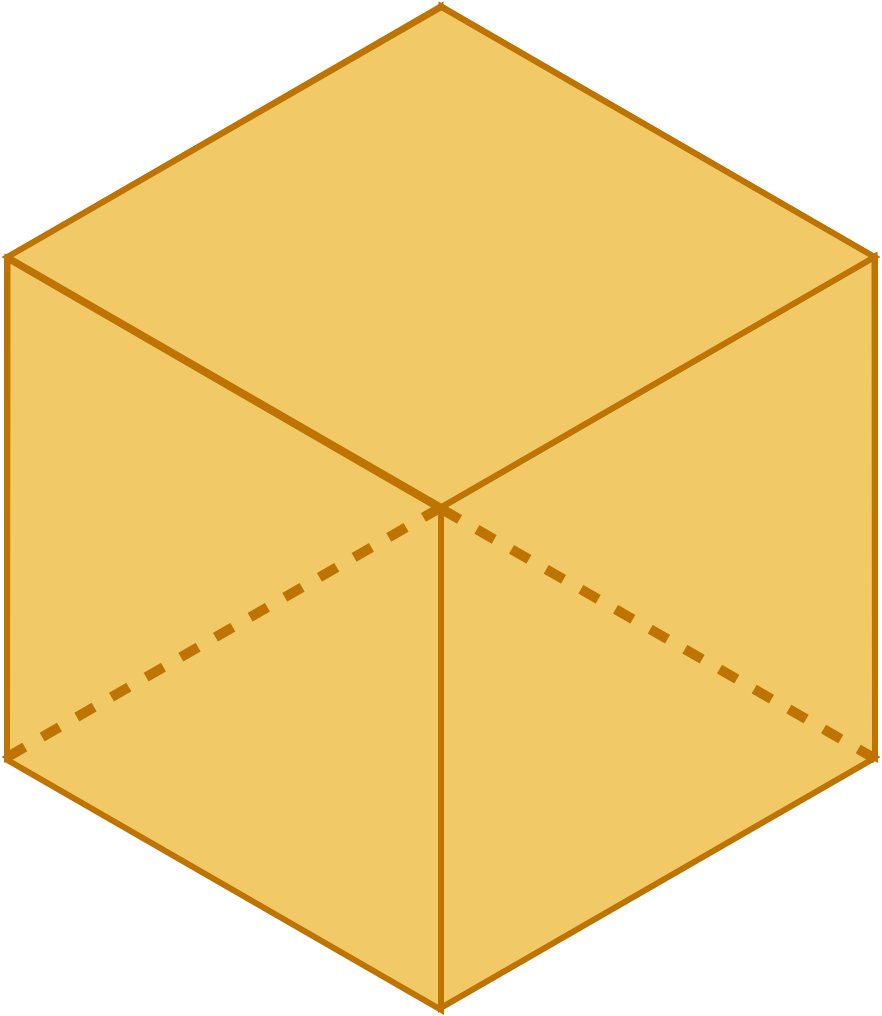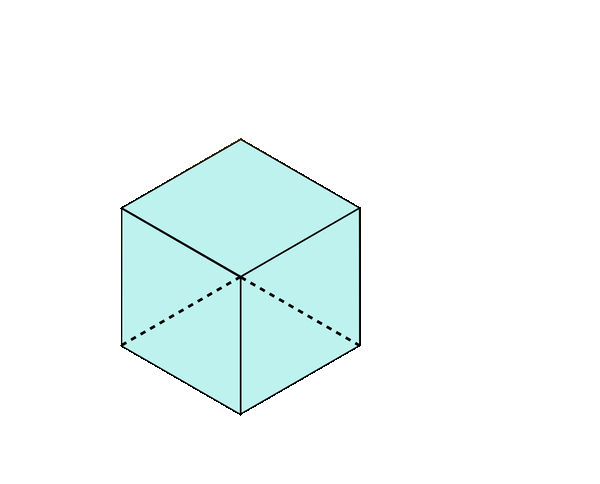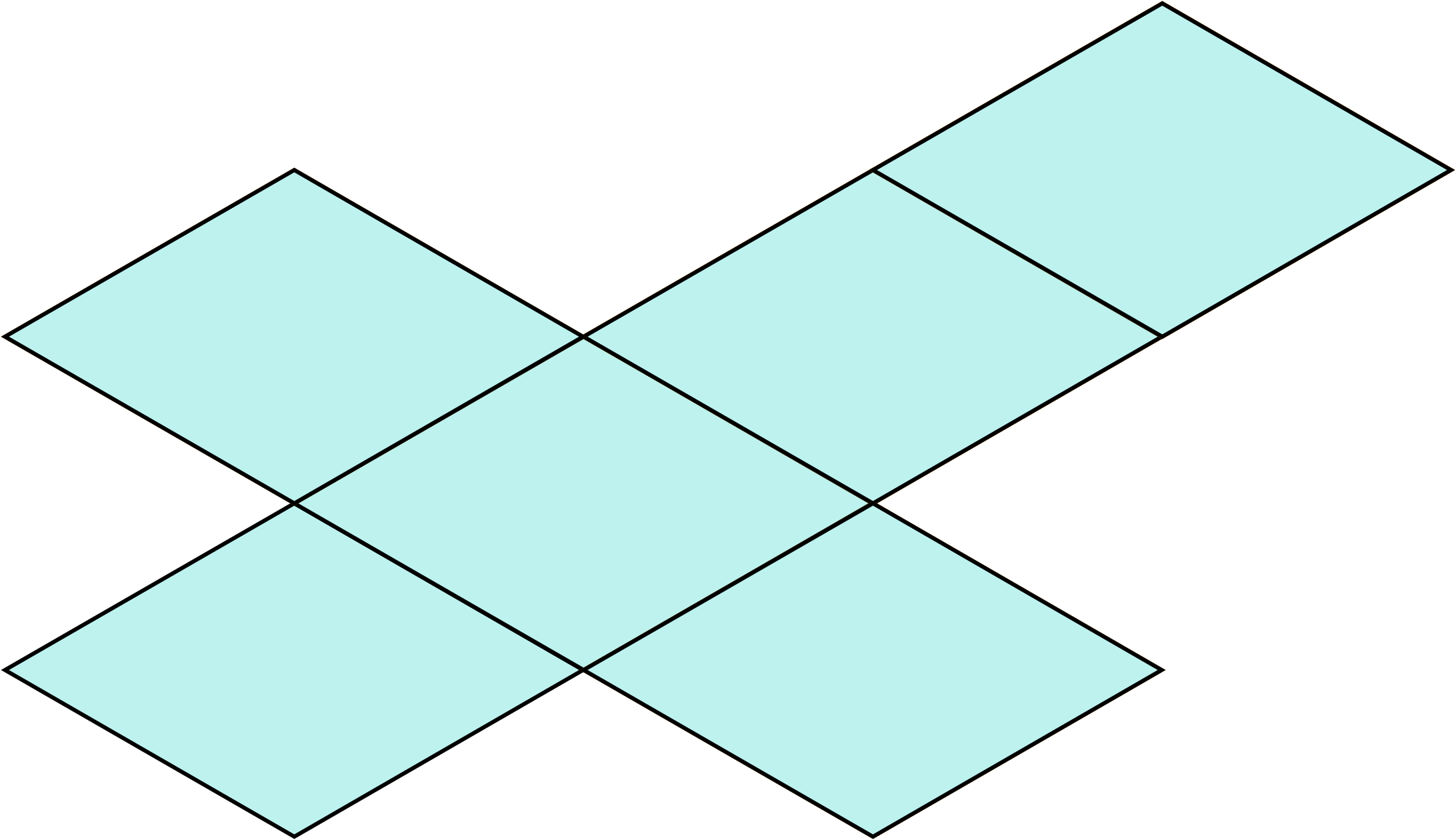When we “unwrap” a 3D shape, we get what we call a net. A surface area net shows us the different 2D shapes that make up the faces of the 3D shape.
We can then find the area of each face and then add them all together to find the total surface area.
Check out our
Calculator
or explore our
Lesson
and
Practice

CALCULATOR

## Surface Area of Cubes & Rectangular Prisms Calculator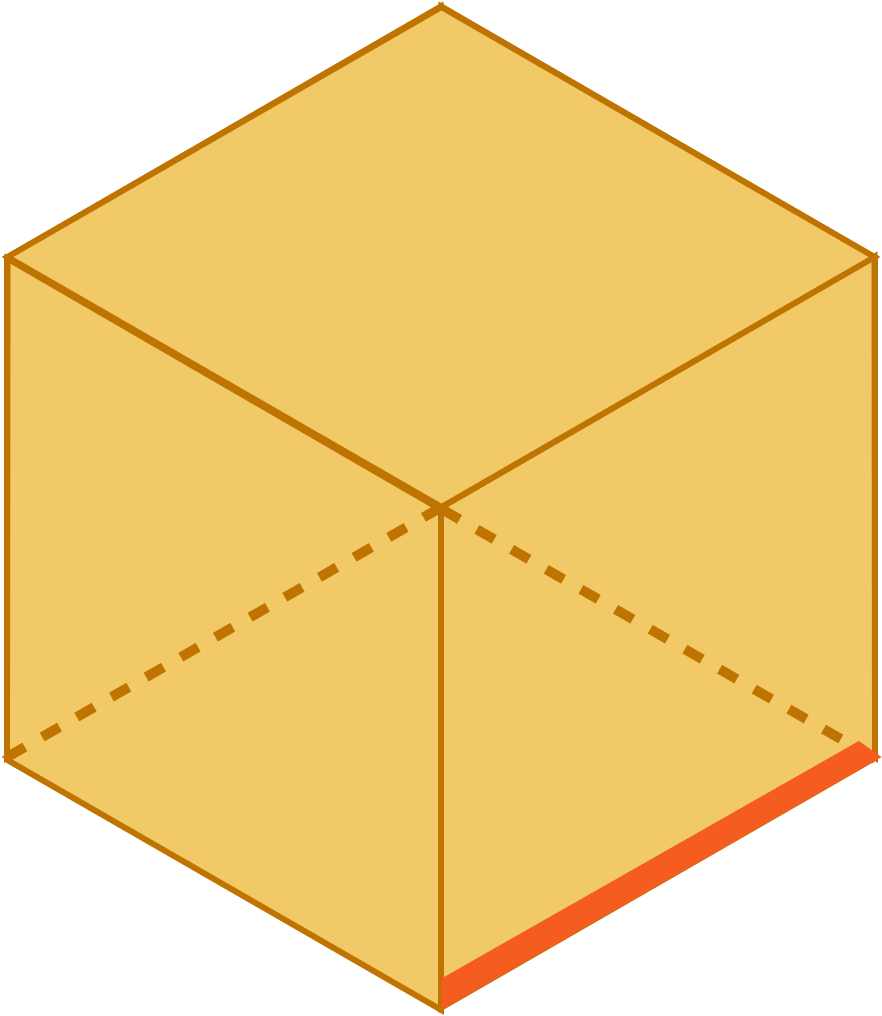KEY STEPS

## How to Find Surface Area of Cubes and Rectangular Prisms

Shape

### Step 1. Unwrap the cube.### Step 2. Calculate the area of each part of the net that makes up the cube.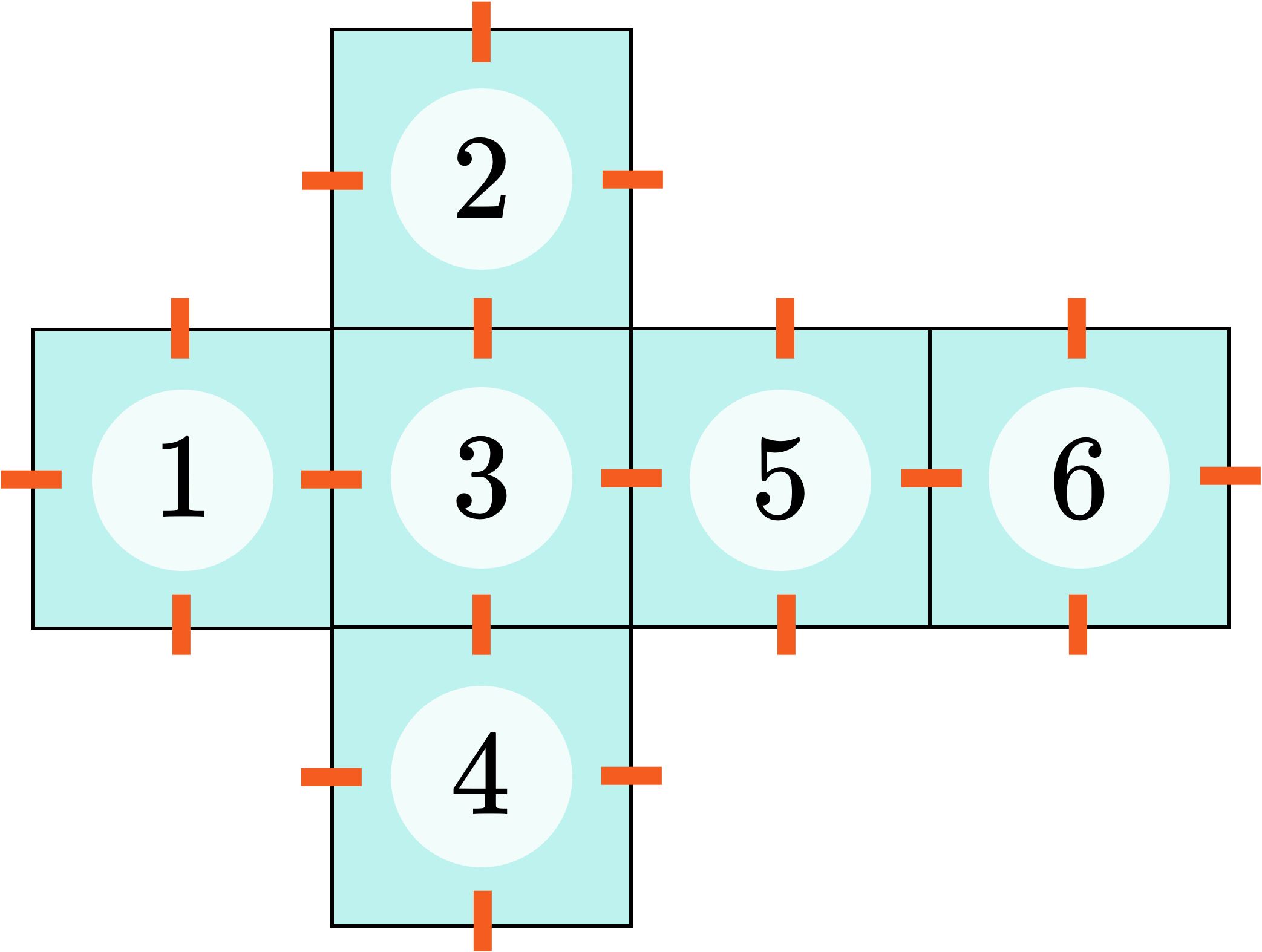Squares 1 - 6

### Step 3. Add up the areas of the shapes that make up the cube.

LESSON
Surface Area of Cubes

## Surface Area of Cubes

The surface area of a cube is equal to the sum of the areas of all the faces (sides) of the cube. If we unwrap a cube, we can see that the net is made up of identical squares.So, we only need to find the area of one of the squares (), and add it times (or multiply it by ) to get the total surface area!Let's unwrap some more cubes to find their surface area!
PRACTICE
Surface Area of Cubes

## Practice: Surface Area of Cubes

Question 1 of 5: Find the surface area of this cube:### Step 1. Unwrap the cube.LESSON
Surface Area of Rectangular Prisms

## Surface Area of Rectangular Prisms

The surface area of a rectangular prism is equal to the sum of the areas of all the faces (sides) of the rectangular prism.
If we unwrap a rectangular prism, we can see that the net is made up of pairs of identical rectangles.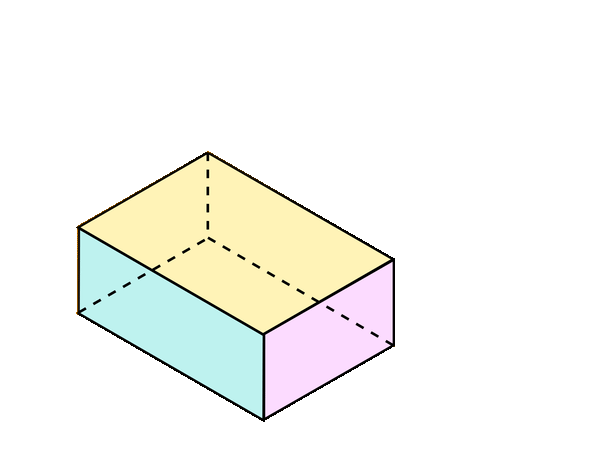So, we only need to find the area of one rectangle from each pair. Then we double each of the three rectangle areas and add them together!
Let’s unwrap some more rectangular prisms and find their total surface areas!
PRACTICE
Surface Area of Rectangular Prisms

## Practice: Surface Area of Rectangular Prisms

Question 1 of 5: Find the surface area of this rectangular prism: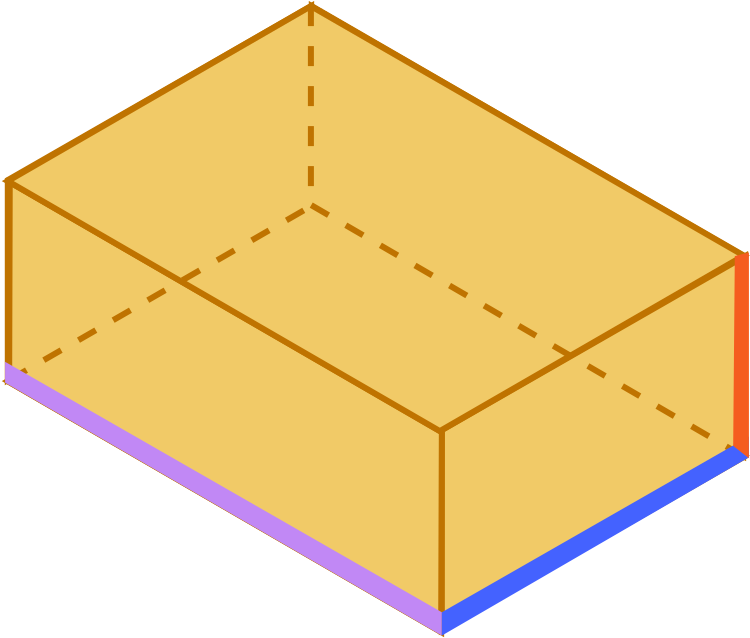### Step 1. Unwrap the rectangular prism.CONCLUSION
Nice work, look at you go! Thanks for checking out this lesson ☺️🙏. Where to next?Leave Feedback